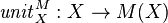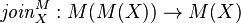While reading through this week’s Issue 160 – December 1, 2010, of the “Haskell Weekly News,” by Daniel Santa Cruz, on the Haskell-Cafe mailing list, I came across the following interesting blog entry describing a reading list for “mathematically-impaired computer scientists trying to learn category theory”:

Category theory for Haskell programmers | Geoff Hulette

In this list, Hulette describes himself as “a computer scientist and Haskell programmer without a strong background in advanced math.”  Now, I am always interested in writings on category theory for students of Haskell who are not necessarily mathematicians.

He started out by recommending Brent Yorgey’s “Typeclassopedia.”  While I can understand the motivation for beginning a reading list with a type class description, and have much respect for Brent Yorgey, I would have preferred to have this reference provided later in the list, and instead begun the list with a more elementary introduction to category theory per se, perhaps along the lines of Conceptual Mathematics: A First Introduction to Categories, by F. William Lawvere and Stephen H. Schanuel (of which I have a copy of the first edition).  The main problems with Lawvere’s book are that it is not related to Haskell, and proceeds somewhat too slowly (for me).  Hulette does provide a reference to this book, but only at the end of his reading list.

Although Hulette does provide a reference to Benjamin Pierce’s Basic Category Theory for Computer Scientists, he neglects to provide one for the book Category Theory, by Steve Awodey.  Awodey’s book has been highly recommended as an introductory book for category theory, and probably should be included in any such list.

Hulette does, however, provide an interesting reference for a collection of tutorial videos on category theory by Eugenia Cheng.  I had not known of these videos before, and it can often be helpful to supplant written material with audio/visual supplements.

Hulette also recommends browsing through the category-extras package on Hackage, and reading the type annotations to provide intuition.

But then he includes a suggestion to look at Saunders Mac Lane’s Categories for the Working Mathematician.  Although frequently mentioned as the definitive text on category theory, the publication, as the title indicates, is intended for “the working mathematician”; as such, it may not really be suitable for “mathematically-impaired computer scientists.”  I deliberately left that title out of my own reading list, and was surprised to find it included in a reading list for non-mathematicians.

As of this writing, there have been nine comments added to Hulette’s post, some of which provide valuable references.  I would recommend taking a look at Hulette’s post and the follow-up comments.

If anybody else has any other suggestions on writings for non-mathematicans learning category theory in order to help in learning Haskell, please feel free to leave a comment!

Addendum of Monday, December 6, 2010, at 01:42 AM:

The user solrize has offered the following addition to this list:

Haskell/Category theory – Wikibooks, collection of open-content textbooks

The above Wikibooks site provides an “intuitive feel for what the concepts of category theory are and how they relate to Haskell.”  Topics described include an introduction to categories, followed by descriptions of functors, monads, and the monad laws and their importance.  Exercises are included, and drawings illustrate category theory concepts.  The site introduces Hask, the Haskell category, and later describes functors on Hask, followed by a brief translation of categorical concepts into Haskell.

Of special interest in this site is the description of the origin of Haskell monads in category theory.  Monads tend to be rather difficult to understand when approached in Haskell; however, category theory can help to clarify the concept.  In particular,  the site ties together the category theoretical definition of a monad with the corresponding Haskell definition as follows (quoted from the site):

A monad is a functor, along with two morphisms for every object X in C:

••When the monad under discussion is obvious, we’ll leave out the M superscript for these functions and just talk about unitX and joinX for some X.

Let’s see how this translates to the Haskell typeclass Monad, then.

class Functor m => Monad m where
return :: a -> m a
(>>=)  :: m a -> (a -> m b) -> m b

The class constraint of Functor m ensures that we already have the functor structure: a mapping of objects and of morphisms. return is the (polymorphic) analogue to unitX for any X. But we have a problem. Although return’s type looks quite similar to that of unit, (>>=) bears no resemblance to join. The monad function join :: Monad m => m (m a) -> m a does however look quite similar. Indeed, we can recover join and (>>=) from each other:

join :: Monad m => m (m a) -> m a
join x = x >>= id

(>>=) :: Monad m => m a -> (a -> m b) -> m b
x >>= f = join (fmap f x)

So specifying a monad’s return and join is equivalent to specifying its return and (>>=). It just turns out that the normal way of defining a monad in category theory is to give unit and join, whereas Haskell programmers like to give return and (>>=). Often, the categorical way makes more sense. Any time you have some kind of structure M and a natural way of taking any object X into M(X), as well as a way of taking M(M(X)) into M(X), you probably have a monad.

This kind of correspondence between category theoretical monads and Haskell monads helps to clarify the concept of a monad in Haskell. Whereas

class Functor m => Monad m where
return :: a -> m a
(>>=)  :: m a -> (a -> m b) -> m b

by itself can be confusing,

••• together with

join :: Monad m => m (m a) -> m a
join x = x >>= id

(>>=) :: Monad m => m a -> (a -> m b) -> m b
x >>= f = join (fmap f x)

can be less confusing because join and (>>=) can be recovered from each other.

1.Don’t you know about

•